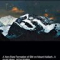Class Central is learner-supported. When you buy through links on our site, we may earn an affiliate commission.

# Matlab Programming for Numerical Computation

This course may be unavailable.

### Overview

MATLAB is a popular language for numerical computation. This course introduces students to MATLAB programming, and demonstrate it’s use for scientific computations. The basis of computational techniques are expounded through various coding examples and problems, and practical ways to use MATLAB will be discussed.

The objective of this course is to introduce undergraduate students to computational methods using MATLAB. At the end of this course, a student would:

Learn basics of MATLAB programming

• Get introduced to numerical methods for engineering problems
• Will be able to use MATLAB to solve computational problems

### Syllabus

Module 1: Introduction to MATLAB Programming
This module will introduce the students to MATLAB programming through a few examples. Students who have used MATLAB are still recommended to do this module, as it introduces MATLAB in context of how we use it in this course
Lecture 1-1 Basics of MATLAB programming
Lecture 1-2 Array operations in MATLAB
Lecture 1-3 Loops and execution control
Lecture 1-4 Working with files: Scripts and Functions
Lecture 1-5 Plotting and program output

Module 2: Approximations and Errors
Taylor’s / Maclaurin series expansion of some functions will be used to introduce approximations and errors in computational methods
Lecture 2-1 Defining errors and precision in numerical methods
Lecture 2-2 Truncation and round-off errors
Lecture 2-3 Error propagation, Global and local truncation errors

Module 3: Numerical Differentiation and Integration
Methods of numerical differentiation and integration, trade-off between truncation and round-off errors, error propagation and MATLAB functions for integration will be discussed.
Lecture 3-1 Numerical Differentiation in single variable
Lecture 3-2 Numerical differentiation: Higher derivatives
Lecture 3-3 Differentiation in multiple variables
Lecture 3-4 Newton-Cotes integration formulae
Lecture 3-5 Multi-step application of Trapezoidal rule
Lecture 3-6 MATLAB functions for integration

Module 4: Linear Equations
The focus of this module is to do a quick introduction of most popular numerical methods in linear algebra, and use of MATLAB to solve practical problems.
Lecture 4-1 Linear algebra in MATLAB
Lecture 4-2 Gauss Elimination
Lecture 4-3 LU decomposition and partial pivoting
Lecture 4-4 Iterative methods: Gauss Siedel
Lecture 4-5 Special Matrices: Tri-diagonal matrix algorithm

Module 5: Nonlinear Equations
After introduction to bisection rule, this module primarily covers Newton-Raphson method and MATLAB routines fzero and fsolve.
Lecture 5-1 Nonlinear equations in single variable
Lecture 5-2 MATLAB function fzero in single variable
Lecture 5-3 Fixed-point iteration in single variable
Lecture 5-4 Newton-Raphson in single variable
Lecture 5-5 MATLAB function fsolve in single and multiple variables
Lecture 5-6 Newton-Raphson in multiple variables

Module 6: Regression and Interpolation
The focus will be practical ways of using linear and nonlinear regression and interpolation functions in MATLAB.
Lecture 6-1 Introduction
Lecture 6-2 Linear least squares regression(including lsqcurvefit function)
Lecture 6-3 Functional and nonlinear regression (including lsqnonlin function)
Lecture 6-4 Interpolation in MATLAB using spline and pchip

Module 7: Ordinary Differential Equations (ODE) – Part 1
Explicit ODE solving techniques in single variable will be covered in this module.
Lecture 7-1 Introduction to ODEs; Implicit and explicit Euler’s methods
Lecture 7-2 Second-Order Runge-Kutta Methods
Lecture 7-3 MATLAB ode45 algorithm in single variable
Lecture 7-4 Higher order Runge-Kutta methods
Lecture 7-5 Error analysis of Runge-Kutta method

Module 8: Ordinary Differential Equations (ODE) – Practical aspects
This module will cover ODE solving in multiple variables, stiff systems, and practical problems. The importance of ODEs in engineering is reflected by the fact that two modules are dedicated to ODEs.
Lecture 8-1 MATLAB ode45 algorithm in multiple variables
Lecture 8-2 Stiff ODEs and MATLAB ode15s algorithm
Lecture 8-3 Practical example for ODE-IVP
Lecture 8-4 Solving transient PDE using Method of Lines

Niket Kaisare

## Reviews

5.0 rating, based on 1 Class Central review

Start your review of Matlab Programming for Numerical Computation

•Sandeep Chatterjee
Very useful course. Step by Step learning process , from week 1 of beginning, they teach plotting, working with vectors, and algorithms.

Sir is awesome.

### Never Stop Learning.

Get personalized course recommendations, track subjects and courses with reminders, and more.